Quality and Process Methods > Control Chart Builder > Statistical Details for Control Chart Builder > Control Limits for Individual Measurement and Moving Range Charts
Publication date: 08/13/2020

## Control Limits for Individual Measurement and Moving Range Charts

Control limits for Individual Measurement charts are computed as follows.

LCL for Individual Measurement Chart =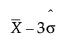UCL for Individual Measurement Chart =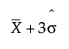Control limits for Individual Measurement charts with sigma estimated by the median moving range are computed as follows.

LCL for Individual Measurement Chart =UCL for Individual Measurement Chart =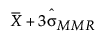Control limits for Moving Range charts are computed as follows.

LCL for Moving Range Chart = 0

UCL for Moving Range Chart =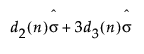Control limits for Median Moving Range charts are computed as follows.

LCLMMR = max(0, MMR - kd3(n)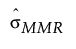)

UCLMMR = MMR + kd3(n)The standard deviation for Individual Measurement and Moving Range charts is estimated by: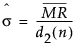The standard deviation for Individual Measurement and Moving Range charts when using the median is estimated by:= MMR/0.954

where: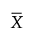= the mean of the individual measurements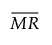= the mean of the nonmissing moving ranges computed as (MR2+MR3+...+MRN)/(N-1) where MRi = |xi - xi-1|.

MMR = the median of the nonmissing moving ranges

σ = the process standard deviation

d2(n) = expected value of the range of n independent normally distributed variables with unit standard deviation.

d3(n) = standard deviation of the range of n independent normally distributed variables with unit standard deviation

Note: Moving Range charts in Control Chart Builder use a range span of n=2.